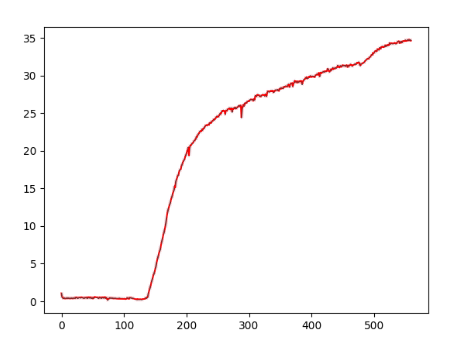# Is there a reason for KL divergence to increase?

I am using Variational Autoencoder(VAE) and KL divergence with recon loss as criteria.
Interestingly after 100 epochs or so, KL divergence starts to increase exponentially.If I understand correctly shouldn’t it decrease rather than an increase in such a manner?
The code I am using as below:

``````    def kl_divergence(z, mu, std):
p = torch.distributions.Normal(torch.zeros_like(mu), torch.ones_like(std))
q = torch.distributions.Normal(mu, std)
log_qzx = q.log_prob(z)
log_pz = p.log_prob(z)
kl = (log_qzx - log_pz)
kl = kl.sum(-1)
return kl
``````

where `z` is 1D spectrum data.

The same also observed using `torch.distributions.kl_divergence’

``````    def kl_divergence(self, z, mu, std):
p = torch.distributions.Normal(torch.zeros_like(mu), torch.ones_like(std))
q = torch.distributions.Normal(mu, std)
kl_t = torch.distributions.kl_divergence(q, p).sum(-1)
return kl_t
``````